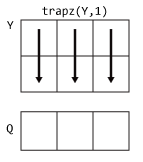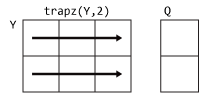# trapz

Trapezoidal numerical integration

## Syntax

``Q = trapz(Y)``
``Q = trapz(X,Y)``
``Q = trapz(___,dim)``

## Description

example

````Q = trapz(Y)` computes the approximate integral of `Y` via the trapezoidal method with unit spacing. The size of `Y` determines the dimension to integrate along:If `Y` is a vector, then `trapz(Y)` is the approximate integral of `Y`.If `Y` is a matrix, then `trapz(Y)` integrates over each column and returns a row vector of integration values.If `Y` is a multidimensional array, then `trapz(Y)` integrates over the first dimension whose size does not equal 1. The size of this dimension becomes 1, and the sizes of other dimensions remain unchanged.```

example

````Q = trapz(X,Y)` integrates `Y` with respect to the coordinates or scalar spacing specified by `X`. If `X` is a vector of coordinates, then `length(X)` must be equal to the size of the first dimension of `Y` whose size does not equal 1.If `X` is a scalar spacing, then `trapz(X,Y)` is equivalent to `X*trapz(Y)`. ```

example

````Q = trapz(___,dim)` integrates along the dimension `dim` using any of the previous syntaxes. You must specify `Y`, and optionally can specify `X`. If you specify `X`, then it can be a scalar or a vector with length equal to `size(Y,dim)`. For example, if `Y` is a matrix, then `trapz(X,Y,2)` integrates each row of `Y`.```

## Examples

collapse all

Calculate the integral of a vector where the spacing between data points is 1.

Create a numeric vector of data.

`Y = [1 4 9 16 25];`

`Y` contains function values for $f\left(x\right)={x}^{2}$ in the domain [1, 5].

Use `trapz` to integrate the data with unit spacing.

`Q = trapz(Y)`
```Q = 42 ```

This approximate integration yields a value of `42`. In this case, the exact answer is a little less, $41\frac{1}{3}$. The `trapz` function overestimates the value of the integral because f(x) is concave up.

Calculate the integral of a vector where the spacing between data points is uniform, but not equal to 1.

Create a domain vector.

`X = 0:pi/100:pi;`

Calculate the sine of `X`.

`Y = sin(X);`

Integrate `Y` using `trapz`.

`Q = trapz(X,Y)`
```Q = 1.9998 ```

When the spacing between points is constant, but not equal to 1, an alternative to creating a vector for `X` is to specify the scalar spacing value. In that case, `trapz(pi/100,Y)` is the same as `pi/100*trapz(Y)`.

Integrate the rows of a matrix where the data has a nonuniform spacing.

Create a vector of x-coordinates and a matrix of observations that take place at the irregular intervals. The rows of `Y` represent velocity data, taken at the times contained in `X`, for three different trials.

```X = [1 2.5 7 10]; Y = [5.2 7.7 9.6 13.2; 4.8 7.0 10.5 14.5; 4.9 6.5 10.2 13.8];```

Use `trapz` to integrate each row independently and find the total distance traveled in each trial. Since the data is not evaluated at constant intervals, specify `X` to indicate the spacing between the data points. Specify `dim = 2` since the data is in the rows of `Y`.

`Q1 = trapz(X,Y,2)`
```Q1 = 3×1 82.8000 85.7250 82.1250 ```

The result is a column vector of integration values, one for each row in `Y`.

Create a grid of domain values.

```x = -3:.1:3; y = -5:.1:5; [X,Y] = meshgrid(x,y);```

Calculate the function $f\left(x,y\right)={x}^{2}+{y}^{2}$ on the grid.

`F = X.^2 + Y.^2;`

`trapz` integrates numeric data rather than functional expressions, so in general the expression does not need to be known to use `trapz` on a matrix of data. In cases where the functional expression is known, you can instead use `integral`, `integral2`, or `integral3`.

Use `trapz` to approximate the double integral

`$I={\int }_{-5}^{5}{\int }_{-3}^{3}\left({x}^{2}+{y}^{2}\right)dx\phantom{\rule{0.2222222222222222em}{0ex}}dy$`

To perform double or triple integrations on an array of numeric data, nest function calls to `trapz`.

`I = trapz(y,trapz(x,F,2))`
```I = 680.2000 ```

`trapz` performs the integration over x first, producing a column vector. Then, the integration over y reduces the column vector to a single scalar. `trapz` slightly overestimates the exact answer of 680 because f(x,y) is concave up.

## Input Arguments

collapse all

Numeric data, specified as a vector, matrix, or multidimensional array. By default, `trapz` integrates along the first dimension of `Y` whose size does not equal 1.

Data Types: `single` | `double`
Complex Number Support: Yes

Point spacing, specified as `1` (default), a uniform scalar spacing, or a vector of coordinates.

• If `X` is a scalar, then it specifies a uniform spacing between the data points and `trapz(X,Y)` is equivalent to `X*trapz(Y)`.

• If `X` is a vector, then it specifies x-coordinates for the data points and `length(X)` must be the same as the size of the integration dimension in `Y`.

Data Types: `single` | `double`

Dimension to operate along, specified as a positive integer scalar. If you do not specify the dimension, then the default is the first array dimension of size greater than 1.

Consider a two-dimensional input array, `Y`:

• `trapz(Y,1)` works on successive elements in the columns of `Y` and returns a row vector.• `trapz(Y,2)` works on successive elements in the rows of `Y` and returns a column vector.If `dim` is greater than `ndims(Y)`, then `trapz` returns an array of zeros of the same size as `Y`.

collapse all

### Trapezoidal Method

`trapz` performs numerical integration via the trapezoidal method. This method approximates the integration over an interval by breaking the area down into trapezoids with more easily computable areas. For example, here is a trapezoidal integration of the sine function using eight evenly-spaced trapezoids:For an integration with `N+1` evenly spaced points, the approximation is

`$\begin{array}{c}\underset{a}{\overset{b}{\int }}f\left(x\right)dx\text{\hspace{0.17em}}\text{\hspace{0.17em}}\approx \text{\hspace{0.17em}}\text{\hspace{0.17em}}\frac{b-a}{2N}\sum _{n=1}^{N}\left(f\left({x}_{n}\right)+f\left({x}_{n+1}\right)\right)\\ =\frac{b-a}{2N}\left[f\left({x}_{1}\right)+2f\left({x}_{2}\right)+...+2f\left({x}_{N}\right)+f\left({x}_{N+1}\right)\right]\text{\hspace{0.17em}}\text{\hspace{0.17em}},\end{array}$`

where the spacing between each point is equal to the scalar value $\frac{b-a}{N}$. By default MATLAB® uses a spacing of 1.

If the spacing between the `N+1` points is not constant, then the formula generalizes to

`$\underset{a}{\overset{b}{\int }}f\left(x\right)dx\text{\hspace{0.17em}}\text{\hspace{0.17em}}\approx \text{\hspace{0.17em}}\text{\hspace{0.17em}}\text{\hspace{0.17em}}\frac{1}{2}\sum _{n=1}^{N}\left({x}_{n+1}-{x}_{n}\right)\left[f\left({x}_{n}\right)+f\left({x}_{n+1}\right)\right]\text{\hspace{0.17em}},$`

where $a={x}_{1}<{x}_{2}<\text{\hspace{0.17em}}\text{\hspace{0.17em}}...\text{\hspace{0.17em}}\text{\hspace{0.17em}}<{x}_{N}<{x}_{N+1}=b$, and $\left({x}_{n+1}-{x}_{n}\right)$ is the spacing between each consecutive pair of points.

## Tips

• Use `trapz` and `cumtrapz` to perform numerical integrations on discrete data sets. Use `integral`, `integral2`, or `integral3` instead if a functional expression for the data is available.

• `trapz` reduces the size of the dimension it operates on to 1, and returns only the final integration value. `cumtrapz` also returns the intermediate integration values, preserving the size of the dimension it operates on.

## Version History

Introduced before R2006a